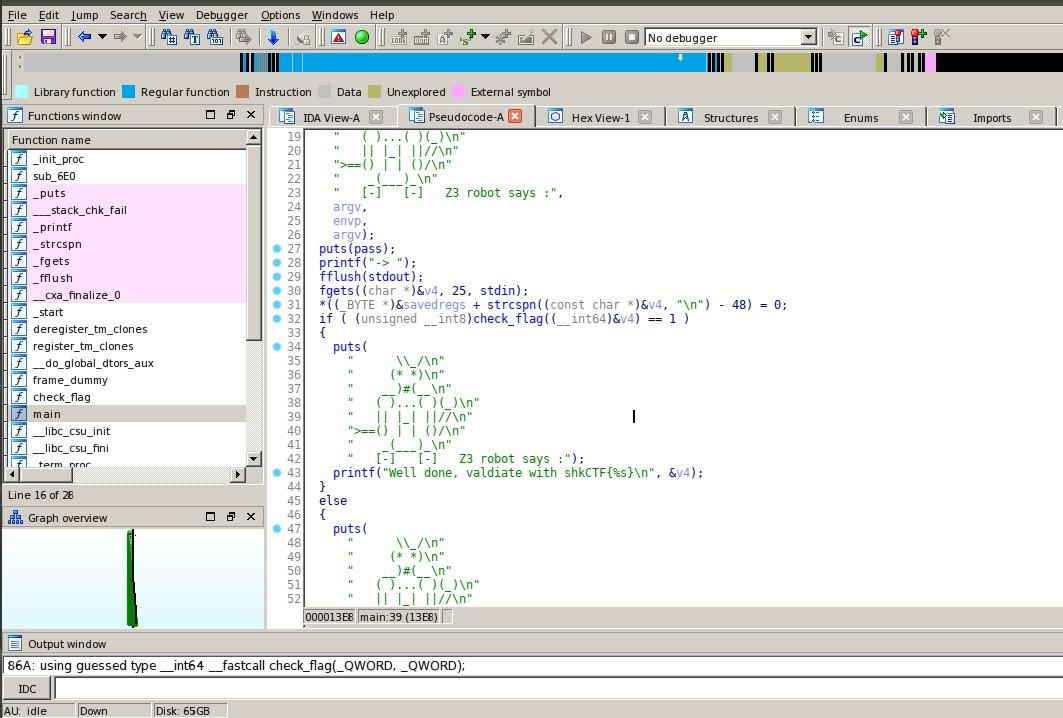# Z3 API in Python¶

Z3 is a state-of-the art theorem prover from Microsoft Research. It is a low level tool. It is best used as a component in the context of other tools that require solving logical formulas over one or more theories. Here we introduce how to use Z3 effectively for logical modeling and solving.

#### Installation¶

pip install z3-solver

#### Z3 for CTFs¶

Z3 Can be used in solving ctf challenges which involves solving arithmetic equations that can't be solved manually.

##### Basic solve¶

To solve most reversing challenges involving solving equations, it involves few concepts which might be new to you.

Lets begin with a simple solving of equations

x+2*y=3
3*x-y=2


The first step is initialization of variables x and y

x=Int('x')
y=Int('y')

Then we create a general purpose solver s.

s=Solver()

Adds a constraint to a Solver s which we can get from the binary.

s.add(x + 2*y==3)


Next we need to checks if the solution exists for the given equation It Returns sat if the solution exist, else if, the solver cannot find a satisfying assignment, then it will return unsat

s.check()

Then Get the satisfying assignment, which will only work if s.check() gives sat

s.model()

from z3 import *
x=Int('x')
y=Int('y')
s=Solver()
s.check()
while(s.check()==sat):
print(s.model()[x])
print(s.model()[y])

##### Bit-Vectors¶

To create bit-vector variables and constants. The function BitVec('x', 32) creates a bit-vector variable in Z3 named x with 32 bits.

x = BitVec('x', 32)
y = BitVec('y', 32)


from z3 import *
a1=BitVec('x',32)
a2=BitVec('y',32)
s.check()
val=s.model()
print(val)

##### Boolean formula¶

To write a boolean formula, we will need to declare boolean variables for the solver. You can do this in Python using the Bool().

###### Few boolean functions¶
• Or() - Asserts at least one argument given is true.
• Distinct() - Asserts that all given variables are distinct.
• Sum() - Creates a variable which is equal to the sum of the arguments.
• Product() - Creates a variable equal to the product of the arguments.
• And() - Asserts that all arguments given are true.
from z3 import *
x = Bool("x")
y = Bool("y")
x_or_y = Or([x,y])
x_and_y = And([x,y])
s = Solver() # create a solver s
s.check()
s.model()

##### Sample solve¶

Now let's try out a Sample challenge which involves use of Z3 Binary here

Let's open the binary in ida to view the codeWe notice a function Check_flag which checks the string you gave as the input.

If you go deep into that function you will see many numbers of equations used to check each of the characters in our string. This can be used for finding the actual input required. As you may have noticed , there are too many equations which makes it impossible to solve manually. This is where we use our concept of Z3. Solve each of these equations using z3 in python and find the string the program actually needs.

Solution here

Which will give us our output flag !!!

Practice Challenges